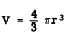Custom SearchSPHERES A SPHERE is a solid figure with all points on its surface equally distant from its center.Figure 18-19.-Parts of a sphere. In figure 18-19, the center of the sphere is point 0.  A RADIUS of a sphere is a straight line segment joining the center of the sphere to a point on the surface. Lines OA, OB, OC, OD, OE, and OF in figure 18-19 are radii. A DIAMETER of a sphere is a straight line segment joining two points on the surface and passing through the center of the sphere. Lines AB, CD, and EF in figure 18-19 are diameters. A HEMISPHERE is half of a sphere. Circles of various sizes may be drawn on the surface of a sphere. The largest circle that may be so drawn is one with a radius equal to the radius of the sphere. Such a circle is a GREAT CIRCLE. In figure 18-19, circles AEBF, ACBD, and CEDF are great circles.  On the surface of a sphere, the shortest distance between two points is an arc of a great circle drawn so that it passes through the two points. This explains the importance of great circles in the science of navigation, since the earth is approximately a sphere. Surface Area The surface area of a sphere may be calculated by multiplying 4 times ‘II times the square of the radius. Written as a formula, this is A = 4pr2 The formula for the surface area of a sphere may be rewritten as follows: A = (2nr)(2r) When the formula is factored in this way, it is easy to see that the surface area of a sphere is simply its circumference times its diameter. Volume The volume of a sphere whose radius is r is given by the formulaEXAMPLE: Find the volume of a sphere whose diameter is 42 inches. SOLUTION:Practice problems. Calculate the surface area and the volume of the sphere in each of the following problems: 1. Radius = 7 inches  2. Radius = 14 cm Answers: 1. Area = 615 sq in. (approx.) Volume = 1436 cu in. (approx.) 2. Area = 2462 sq cm (approx.) Volume = 11,489 cu cm (approx.)